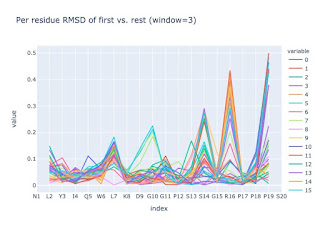Per residue RMSD

## Wednesday 7 July 2021

### Per residue RMSD

Recently I calculated the local RMSD caused by each residue and I thought I'd share the methods I used using PyRosetta —it is nothing at all novel, but I could not find a suitable implementation. The task is simple given two poses, find out what residue's backbone is changing the most by scanning along comparing each a short peptide window from each.

#### Premise

First, I should premise that this is the most basic approach and contact area difference (CAD) is actually preferred, but for some tasks it is nice to keep it simple.

#### Model

A key part of science is doing appropriate controls. A lovely control for this is the tryptophan-cage mini protein NMR structure (PDB:1L2Y), because it is very small and has thirty-something poses.

Loading a multi-model structure in PyRosetta is rather bizarre as it gets loaded as one pose —unless there is a secret function I am missing. So it needs to be split:
``````original = pyrosetta.toolbox.rcsb.pose_from_rcsb('1L2Y')
mini = pyrosetta.rosetta.protocols.grafting.return_region(original, 1,20)
nmr = pyrosetta.rosetta.utility.vector1_core_pose_Pose()
n = [pyrosetta.rosetta.protocols.grafting.return_region(original, 1+i,20+i) for i in range(0, original.total_residue(),20)]
nmr.extend(n)``````

#### RMSD

RMSD (root mean square deviation) is a metric in Ångström of how much on average do the atoms positions differ between two conformations (wiki). If the comparison is between a conformation with an imaginary centroid of an ensemble it is called RMSF (fluctuation). This is a very simple metric and gives the most weight to atoms that change the most and does not take into account several things such functional groups etc. for which there are many "shapes-and-colours" metrics. But RMSD is simple and clear.

In `pyrosetta.rosetta.core.scoring` there are several functions that calculate different forms. They do a superposition beforehand, but it is always wise to check.

One can check manually or simply do a test. So lets copy the first pose and translate it:

``````import numpy as np

x, y, z = (10, 0, 0)
rototrans = np.array([[1, 0, 0, x], [0, 1, 0, y],[0, 0, 1, z],[0, 0, 0, 1]])

copy = nmr.clone()
copy.apply_transform(rototrans)

pyrosetta.rosetta.core.scoring.CA_rmsd(nmr, copy, 1, 3),\
pyrosetta.rosetta.core.scoring.CA_rmsd(nmr, copy),\
pyrosetta.rosetta.core.scoring.bb_rmsd_including_O(nmr, copy),\
pyrosetta.rosetta.core.scoring.all_atom_rmsd(nmr, copy),\
pyrosetta.rosetta.core.scoring.all_atom_rmsd_nosuper(nmr, copy)``````

As hoped all bar the last give near zero values —if the rototranslation part seems gibberish, you may want to read up on 4x4 rototranslational matrices: the learning curve is very steep, but worth it.

#### Simple

With `CA_rmsd` one can specify a window and if the poses have the same residue, the code is very simple.

``````def calculate_simple(ref:pyrosetta.Pose, target: pyrosetta.Pose, window: int= 3):
assert (window - 1) % 2 == 0, 'Odd number windows only'
half_window = int((window - 1)/2)
residue_rsmds = [float('nan')] * half_window
for off_i in range(half_window, ref.total_residue() - half_window):
i = off_i + 1
residue_rsmds.append(pyrosetta.rosetta.core.scoring.CA_rmsd(ref, target, i-half_window, i+half_window))
residue_rsmds.extend([float('nan')] * half_window)
return residue_rsmds``````Here I am comparing to the first model because whereas my control is an ensemble, I am interested in comparing conformational switches between two or more poses. If I did care about an ensemble, I could use an averaged pose —generated with ProDy say to get the RMSF for each atom or residue.
Generally, the window size used is 5. But one could use a larger one.

#### Real world

This all nice, howerver, my conformers have different residues due to missing density and I would like all the backbone. So the code becomes instantly more complex, because the PDB residues need to be compared and one has to make sure only the a given chain is compared. As a result slicing the peptide out of the pose based on PDB numbering has to be done.

``````from typing import *

def slice_for_comparison(pose:pyrosetta.Pose,
from_res: Tuple[int, str],
to_res: Tuple[int, str]) -> Union[None, pyrosetta.Pose]:
"""
Returns None is there is missing density in the range or different chains
"""
# convert to pose
from_resi, from_chain = from_res
to_resi, to_chain = to_res
pdb2pose = pose.pdb_info().pdb2pose
from_r = pdb2pose(res=from_resi, chain=from_chain)
to_r = pdb2pose(res=to_resi, chain=to_chain)
# validity checks.
if from_chain != to_chain:  # different chain. user's doing...
return None
elif from_r==0 or to_r == 0: # missing density
return None
elif to_r - from_r != to_resi - from_resi: # gap
return None
else: # slice
return pyrosetta.Pose(pose, from_r, to_r)

def calculate_window(ref:pyrosetta.Pose,
target: pyrosetta.Pose,
from_res: Tuple[int, str],
to_res: Tuple[int, str],
rmsd_fx: Callable= pyrosetta.rosetta.core.scoring.CA_rmsd
):
sliced_ref = slice_for_comparison(ref, from_res, to_res)
sliced_target = slice_for_comparison(target, from_res, to_res)
# from_resi, to_resi are valid only from CA_rmsd, hence the slicing
if sliced_ref is None or sliced_target is None:
return float('nan')
else:
return rmsd_fx(sliced_ref, sliced_target)

def calculate(ref:pyrosetta.Pose,
target: pyrosetta.Pose,
chain: str='A',
window: int= 3,
rmsd_fx: Callable= pyrosetta.rosetta.core.scoring.CA_rmsd):
assert (window - 1) % 2 == 0, 'Odd number windows only'
half_window = int((window - 1)/2)
# get the boundaries of the pose residue indices making that chain.
ref_pdb = ref.pdb_info().number
chain_id = pyrosetta.rosetta.core.pose.get_chain_id_from_chain(chain, ref)
from_resi = ref_pdb(ref.chain_begin(chain_id))
to_resi = ref_pdb(ref.chain_end(chain_id))
# calculate
residue_rsmds = {i: calculate_window(ref,
target,
(i-half_window, chain),
(i+half_window, chain),
rmsd_fx) for i in range(from_resi+half_window, to_resi-half_window+1)}
return residue_rsmds``````

But despite the complexity caused by the slicing per PDB numbering, the method is nice and simple really...

#### NGL

As an added bonus, one can use NGL to show multiple poses, as seen in a previous blog post.

``````import nglview as nv
from io import StringIO
import time

class ModNGLWidget(nv.NGLWidget):
buffer = pyrosetta.rosetta.std.stringbuf()
pose.dump_pdb(pyrosetta.rosetta.std.ostream(buffer))
fh = StringIO(buffer.str())